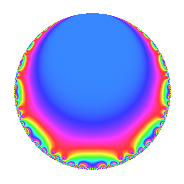# Properties

 Label 8400.2.a.bnLevel $8400$ Weight $2$ Character orbit 8400.a Self dual yes Analytic conductor $67.074$ Analytic rank $1$ Dimension $1$ CM no Inner twists $1$

# Related objects

## Newspace parameters

 Level: $$N$$ $$=$$ $$8400 = 2^{4} \cdot 3 \cdot 5^{2} \cdot 7$$ Weight: $$k$$ $$=$$ $$2$$ Character orbit: $$[\chi]$$ $$=$$ 8400.a (trivial)

## Newform invariants

 Self dual: yes Analytic conductor: $$67.0743376979$$ Analytic rank: $$1$$ Dimension: $$1$$ Coefficient field: $$\mathbb{Q}$$ Coefficient ring: $$\mathbb{Z}$$ Coefficient ring index: $$1$$ Twist minimal: no (minimal twist has level 21) Fricke sign: $$1$$ Sato-Tate group: $\mathrm{SU}(2)$

## $q$-expansion

 $$f(q)$$ $$=$$ $$q + q^{3} - q^{7} + q^{9} + O(q^{10})$$ $$q + q^{3} - q^{7} + q^{9} - 4q^{11} + 2q^{13} + 6q^{17} - 4q^{19} - q^{21} + q^{27} - 2q^{29} - 4q^{33} - 6q^{37} + 2q^{39} + 2q^{41} - 4q^{43} + q^{49} + 6q^{51} - 6q^{53} - 4q^{57} - 12q^{59} - 2q^{61} - q^{63} + 4q^{67} + 6q^{73} + 4q^{77} + 16q^{79} + q^{81} - 12q^{83} - 2q^{87} - 14q^{89} - 2q^{91} - 18q^{97} - 4q^{99} + O(q^{100})$$

## Embeddings

For each embedding $$\iota_m$$ of the coefficient field, the values $$\iota_m(a_n)$$ are shown below.

For more information on an embedded modular form you can click on its label.

Label $$\iota_m(\nu)$$ $$a_{2}$$ $$a_{3}$$ $$a_{4}$$ $$a_{5}$$ $$a_{6}$$ $$a_{7}$$ $$a_{8}$$ $$a_{9}$$ $$a_{10}$$
1.1
 0
0 1.00000 0 0 0 −1.00000 0 1.00000 0
 $$n$$: e.g. 2-40 or 990-1000 Significant digits: Format: Complex embeddings Normalized embeddings Satake parameters Satake angles

## Atkin-Lehner signs

$$p$$ Sign
$$2$$ $$-1$$
$$3$$ $$-1$$
$$5$$ $$1$$
$$7$$ $$1$$

## Inner twists

This newform does not admit any (nontrivial) inner twists.

## Twists

By twisting character orbit
Char Parity Ord Mult Type Twist Min Dim
1.a even 1 1 trivial 8400.2.a.bn 1
4.b odd 2 1 525.2.a.d 1
5.b even 2 1 336.2.a.a 1
12.b even 2 1 1575.2.a.c 1
15.d odd 2 1 1008.2.a.l 1
20.d odd 2 1 21.2.a.a 1
20.e even 4 2 525.2.d.a 2
28.d even 2 1 3675.2.a.n 1
35.c odd 2 1 2352.2.a.v 1
35.i odd 6 2 2352.2.q.e 2
35.j even 6 2 2352.2.q.x 2
40.e odd 2 1 1344.2.a.g 1
40.f even 2 1 1344.2.a.s 1
60.h even 2 1 63.2.a.a 1
60.l odd 4 2 1575.2.d.a 2
80.k odd 4 2 5376.2.c.r 2
80.q even 4 2 5376.2.c.l 2
105.g even 2 1 7056.2.a.p 1
120.i odd 2 1 4032.2.a.k 1
120.m even 2 1 4032.2.a.h 1
140.c even 2 1 147.2.a.a 1
140.p odd 6 2 147.2.e.b 2
140.s even 6 2 147.2.e.c 2
180.n even 6 2 567.2.f.b 2
180.p odd 6 2 567.2.f.g 2
220.g even 2 1 2541.2.a.j 1
260.g odd 2 1 3549.2.a.c 1
280.c odd 2 1 9408.2.a.m 1
280.n even 2 1 9408.2.a.bv 1
340.d odd 2 1 6069.2.a.b 1
380.d even 2 1 7581.2.a.d 1
420.o odd 2 1 441.2.a.f 1
420.ba even 6 2 441.2.e.a 2
420.be odd 6 2 441.2.e.b 2
660.g odd 2 1 7623.2.a.g 1

By twisted newform orbit
Twist Min Dim Char Parity Ord Mult Type
21.2.a.a 1 20.d odd 2 1
63.2.a.a 1 60.h even 2 1
147.2.a.a 1 140.c even 2 1
147.2.e.b 2 140.p odd 6 2
147.2.e.c 2 140.s even 6 2
336.2.a.a 1 5.b even 2 1
441.2.a.f 1 420.o odd 2 1
441.2.e.a 2 420.ba even 6 2
441.2.e.b 2 420.be odd 6 2
525.2.a.d 1 4.b odd 2 1
525.2.d.a 2 20.e even 4 2
567.2.f.b 2 180.n even 6 2
567.2.f.g 2 180.p odd 6 2
1008.2.a.l 1 15.d odd 2 1
1344.2.a.g 1 40.e odd 2 1
1344.2.a.s 1 40.f even 2 1
1575.2.a.c 1 12.b even 2 1
1575.2.d.a 2 60.l odd 4 2
2352.2.a.v 1 35.c odd 2 1
2352.2.q.e 2 35.i odd 6 2
2352.2.q.x 2 35.j even 6 2
2541.2.a.j 1 220.g even 2 1
3549.2.a.c 1 260.g odd 2 1
3675.2.a.n 1 28.d even 2 1
4032.2.a.h 1 120.m even 2 1
4032.2.a.k 1 120.i odd 2 1
5376.2.c.l 2 80.q even 4 2
5376.2.c.r 2 80.k odd 4 2
6069.2.a.b 1 340.d odd 2 1
7056.2.a.p 1 105.g even 2 1
7581.2.a.d 1 380.d even 2 1
7623.2.a.g 1 660.g odd 2 1
8400.2.a.bn 1 1.a even 1 1 trivial
9408.2.a.m 1 280.c odd 2 1
9408.2.a.bv 1 280.n even 2 1

## Hecke kernels

This newform subspace can be constructed as the intersection of the kernels of the following linear operators acting on $$S_{2}^{\mathrm{new}}(\Gamma_0(8400))$$:

 $$T_{11} + 4$$ $$T_{13} - 2$$ $$T_{17} - 6$$ $$T_{19} + 4$$ $$T_{23}$$